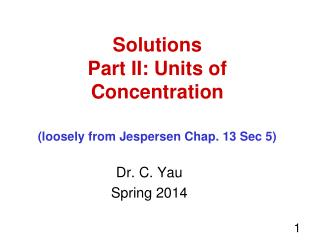DownloadDownload PresentationSolutions Part II: Units of Concentration (loosely from Jespersen Chap. 13 Sec 5)

# Solutions Part II: Units of Concentration (loosely from Jespersen Chap. 13 Sec 5)

Télécharger la présentation## Solutions Part II: Units of Concentration (loosely from Jespersen Chap. 13 Sec 5)

- - - - - - - - - - - - - - - - - - - - - - - - - - - E N D - - - - - - - - - - - - - - - - - - - - - - - - - - -
##### Presentation Transcript

1. SolutionsPart II: Units of Concentration(loosely from Jespersen Chap. 13 Sec 5) Dr. C. Yau Spring 2014

2. Units of Concentration Molarity (M) Molality (m) Mole fraction & mole % Mass %, ppt, ppm, ppb

3. Percent by Mass 1 million = 1,000,000 = 106 1 billion = 1,000,000,000 = 109

4. What does 15% w/w mean? w/w means 15% w/w means 1 ppt means....? 15 ppb means....?

5. Example 13.2 p.598 Please don’t waste time copying the question. You can get it later from your textbook. Seawater is typically 3.5% sea salt and has a density of about 1.03 g mL-1. How many grams of sea salt would be needed to prepare enough seawater solution to completely fill a 62.5 L aquarium? 5

6. Molality vs Molarity Know WHEN m is almost the same as M...under what conditions? If we have a very dilute solution, such as 0.0005 M which is In such a solution, there is very little solute present, therefore 1 L soln = 1 L water. Ans. m = M for very dilute solutions 6

7. Ex 13.3 p.599 An experiment calls for a 0.150 m solution of sodium chloride in water. How many grams of NaCl would have to be dissolved in 500.0 g of water to prepare a solution of this molality?

8. Interconversion of Units of Concentration Example 13.4 p. 601 What is the molality of 10.0% w/w aq NaCl?

9. Example 13.5 p. 602 A certain supply of conc hydrochloric acid has a concentration of 36.0% HCl. The density of the solution is 1.19 g mL-1. Calculate the molar concentration of HCl.

10. p.629 #13.48 What is the molality of NaCl in a solution that is 3.000 M NaCl, with a density of 1.07 g mL-1? Be sure to learn how to convert between different units of concentration. Practice Exer 6-8 on p.600 Exer 9 & 10 on p.602 Exer 11 & 12 on p.603 Do as many as possible: p.629 #48-57.